# Introduction

This is a short tutorial on how to interact with the Soil Data Access (SDA) web-service using R. Queries are written using a dialect of SQL. On first glance SQL appears similar to the language used to write NASIS queries and reports, however, these are two distinct languages. Soil Data Access is a “window” into the spatial and tabular data associated with the current SSURGO snapshot. Queries can contain spatial and tabular filters. If you are new to SDA or SQL, have a look at this page. Here is a SSURGO-specific library of SQL code, organized by task.

Spatial queries can be included in SQL statements submitted to SDA as long as the geometry has first been transformed to WGS84 geographic (or psuedo-Web Mercator) coordinates and formatted as “well known text” (WKT). The `sp` and `rgdal` packages provide functionality for converting between coordinate systems via `spTransform()`. Coordinate reference system definitions (a “CRS”) are typically provided using proj4 notation. You can search for various CRS definitions in a variety of formats using spatialreference.org/.

The `soilDB` library for R provides a helper function (`SDA_query()`) for submitting queries to SDA, processing the result, and reformatting the results into a rectangular table (a `data.frame`). Most of the work required to use the `SDA_query()` function will be writing SQL to describe the columns you would like returned and how the data should be filtered and possibly grouped.

Follow along with the blocks of code below by copying / pasting into a new R “script” document. Each block of command can be run by pasting into the R console, or by “stepping through” lines of code by moving the cursor to the top of a block (in the R script panel) and repeatedly pressing ctrl + enter. Note that `knittr:kable` is used to create cleaner output in the tutorial and isn’t required while following along.

If you are feeling adventurous, have a look at a draft tutorial on queries that return geometry from SDA. Additional tips on advanced spatial queries can be found here.

## Critical Note: SSURGO vs. STATSGO

SSURGO (1:24k soil survey) and STATSGO (1:250k soil survey) records are stored together in SDA. Therefore, it is critical that evey query to SDA include some kind of filter for selecting the appropriate records. Filtering strategies include:

• explicit exclusion of STATSGO records, via `legend.areasymbol != 'US'` in the WHERE clause
• implicit exclusion of STATSGO records, via SSURGO areasymbol in the WHERE clause
• spatial queries using SDA helper functions: e.g. `SDA_Get_Mukey_from_intersection_with_WktWgs84()`
• explicit selection of SSURGO / STATSGO records by record ID: e.g. `mukey`, `cokey`, etc.

Explicit exclusion of STATSGO records:

``````SELECT [...]
FROM legend
INNER JOIN mapunit mu ON mu.lkey = legend.lkey
INNER JOIN component co ON mu.mukey = co.mukey
WHERE legend.areasymbol != 'US' ;``````

Implicit exclusion of STATSGO records:

``````SELECT [...]
FROM legend
INNER JOIN mapunit mu ON mu.lkey = legend.lkey
INNER JOIN component co ON mu.mukey = co.mukey
WHERE legend.areasymbol = 'CA113' ;``````

## Install Required R Packages

You only need to do this once. If you haven’t installed these packages, then copy the code below and paste into the RStudio “console” pane.

``````# run these commands in the R console
# stable version from CRAN + dependencies
install.packages("httr", dep=TRUE)
install.packages("aqp", dep=TRUE)
install.packages("soilDB", dep=TRUE)
install.packages("rgdal", dep = TRUE)
install.packages("raster", dep = TRUE)
install.packages("rgeos", dep = TRUE)``````

# Simple Queries

Now that you have the required packages, load them into the current R session.

``````library(aqp)
library(soilDB)
library(sp)
library(rgdal)
library(raster)
library(rgeos)``````

When was the CA653 survey area last exported to SSURGO?

``SDA_query("SELECT areasymbol, saverest FROM sacatalog WHERE areasymbol = 'CA653'")``
``````##   areasymbol            saverest
## 1      CA653 9/3/2021 5:41:11 PM``````

Are there any survey areas that haven’t been updated since Jan 1, 2010?

``SDA_query("SELECT areasymbol, saverest FROM sacatalog WHERE saverest < '01/01/2010'")``
``## NULL``

What is the most recently updated survey in CA?

``SDA_query("SELECT areasymbol, saverest FROM sacatalog WHERE areasymbol LIKE 'CA%' ORDER BY saverest DESC")[1, ]``
``````##   areasymbol             saverest
## 1      CA804 9/15/2021 8:16:04 PM``````

A simple query from the component table, for a single map unit: `mukey = '461958'`. This is a SSURGO map unit key, therefore STATSGO records are implicitly removed from the results.

``````q <- "SELECT
mukey, cokey, comppct_r, compname, taxclname
FROM component
WHERE mukey = '461958'"

# run the query
res <- SDA_query(q)

# check
mukey cokey comppct_r compname taxclname
461958 21097091 4 Galt NA
461958 21097092 85 San Joaquin Fine, mixed, thermic Abruptic Durixeralfs
461958 21097093 3 Kimball NA
461958 21097094 1 Unnamed NA
461958 21097095 3 Hedge NA
461958 21097096 4 Bruella NA

Get a list of map units that contain “Amador” as minor component. Note that this type of query requires explicit exclusion of STATSGO records.

``````q <- "SELECT
muname, mapunit.mukey, cokey, compname, comppct_r
FROM legend
INNER JOIN mapunit ON mapunit.lkey = legend.lkey
INNER JOIN component on mapunit.mukey = component.mukey
WHERE
-- exclude STATSGO
legend.areasymbol != 'US'
AND majcompflag = 'No'"

# run the query
res <- SDA_query(q)

# check
muname mukey cokey compname comppct_r
Pardee-Ranchoseco complex, 3 to 15 percent slopes 461931 21096986 Amador 3
Pardee cobbly loam, 2 to 15 percent slopes 2924951 21588783 Amador 10
Pardee-Ranchoseco complex, 3 to 15 percent slopes 1420721 21108405 Amador 3
Hicksville sandy clay loam, 0 to 2 percent slopes, occasionally flooded 1612051 21128710 Amador 3
Ultic Haploxeralfs-Mollic Haploxeralfs complex, 3 to 30 percent slopes 2924833 21139852 Amador 5
Mckeonhills clay, 5 to 15 percent slopes 1540892 21622557 Amador 1
``````# optionally save the results to CSV file
# write.csv(res, file='path-to-file.csv', row.names=FALSE)``````

Get basic map unit and component data for a single survey area, Yolo County (CA113). There is no need to exclude STATSGO records because we are specifying a SSURGO areasymbol in the WHERE clause.

``````q <- "SELECT
component.mukey, cokey, comppct_r, compname, taxclname,
taxorder, taxsuborder, taxgrtgroup, taxsubgrp
FROM legend
INNER JOIN mapunit ON mapunit.lkey = legend.lkey
INNER JOIN component ON component.mukey = mapunit.mukey
WHERE legend.areasymbol = 'CA113'"

# run the query
res <- SDA_query(q)

# check
mukey cokey comppct_r compname taxclname taxorder taxsuborder taxgrtgroup taxsubgrp
459208 21463250 85 Balcom Fine-loamy, mixed, thermic Calcixerollic Xerochrepts Inceptisols Ochrepts Xerochrepts Calcixerollic Xerochrepts
459208 21463251 5 Positas NA NA NA NA NA
459208 21463252 5 Sehorn NA NA NA NA NA
459208 21463253 5 Corning NA NA NA NA NA
459209 21463254 85 Balcom Fine-loamy, mixed, thermic Calcixerollic Xerochrepts Inceptisols Ochrepts Xerochrepts Calcixerollic Xerochrepts
459209 21463255 2 Positas NA NA NA NA NA

Cross tabulate the occurrence of components named “Auburn” and “Dunstone” with survey areasymbol. Note that this type of query requires explicit exclusion of STATSGO records.

``````q <- "SELECT areasymbol, component.mukey, cokey, comppct_r, compname, compkind, taxclname
FROM legend
INNER JOIN mapunit ON mapunit.lkey = legend.lkey
INNER JOIN component ON component.mukey = mapunit.mukey
WHERE compname IN ('Auburn', 'Dunstone')
-- exclude STATSGO
AND areasymbol != 'US'
ORDER BY areasymbol, compname"

res <- SDA_query(q)

xtabs(~ areasymbol + compname, data=res)``````
``````##           compname
## areasymbol Auburn Dunstone
##      CA067      9        0
##      CA607     16        0
##      CA612      8       19
##      CA618     29        1
##      CA619     24        1
##      CA620     14        0
##      CA624     24        0
##      CA628     20        0
##      CA630      2        2
##      CA632      2        1
##      CA644     15        1
##      CA648      6        0
##      CA649     13        3
##      CA707     11        0
##      CA731      1        2
##      CA750      0        2``````

# Helper Functions

## fetchSDA

``````library(ggplot2)
library(gridExtra)
library(viridisLite)

# query soil components by areasymbol and musym
test <- fetchSDA(WHERE = "areasymbol = 'IN005' AND musym = 'MnpB2'")

# profile plot
par(mar = c(0, 0, 0, 1))
plotSPC(test)``````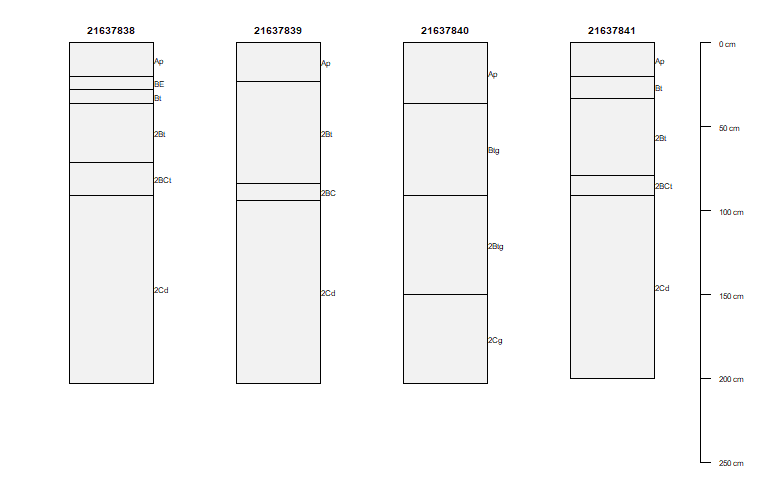``````# convert the data for depth plot
clay_slice <- horizons(slice(test, 0:200 ~ claytotal_l + claytotal_r + claytotal_h))
names(clay_slice) <- gsub("claytotal_", "", names(clay_slice))

om_slice <- horizons(slice(test, 0:200 ~ om_l + om_r + om_h))
names(om_slice) <- gsub("om_", "", names(om_slice))

test2 <- rbind(data.frame(clay_slice, var = "clay"),
data.frame(om_slice, var = "om")
)

h <- merge(test2, site(test)[c("nationalmusym", "cokey", "compname", "comppct_r")],
by = "cokey",
all.x = TRUE
)

# depth plot of clay content by soil component
gg_comp <- function(x) {
ggplot(x) +
geom_line(aes(y = r, x = hzdept_r)) +
geom_line(aes(y = r, x = hzdept_r)) +
geom_ribbon(aes(ymin = l, ymax = h, x = hzdept_r), alpha = 0.2) +
xlim(200, 0) +
xlab("depth (cm)") +
facet_grid(var ~ nationalmusym + paste(compname, comppct_r)) +
coord_flip()
}
g1 <- gg_comp(subset(h, var == "clay"))
g2 <- gg_comp(subset(h, var == "om"))

grid.arrange(g1, g2)``````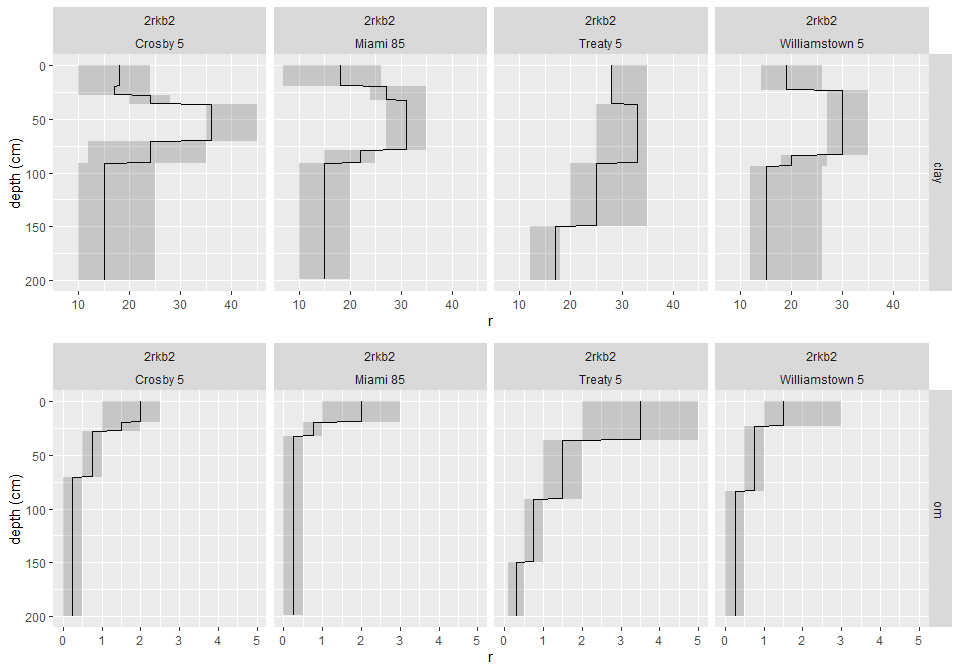``````# query all Miami major components
s <- get_component_from_SDA(WHERE = "compname = 'Miami' \n
AND majcompflag = 'Yes' AND areasymbol != 'US'")

# landform vs 3-D morphometry
test <- {
subset(s, ! is.na(landform) | ! is.na(geompos)) ->.;
split(., .\$drainagecl, drop = TRUE) ->.;
lapply(., function(x) {
test = data.frame()
test = as.data.frame(table(x\$landform, x\$geompos))
test\$compname   = x\$compname
test\$drainagecl = x\$drainagecl
names(test)[1:2] <- c("landform", "geompos")
return(test)
}) ->.;
do.call("rbind", .) ->.;
.[.\$Freq > 0, ] ->.;
within(., {
landform = reorder(factor(landform), Freq, max)
geompos  = reorder(factor(geompos),  Freq, max)
geompos  = factor(geompos, levels = rev(levels(geompos)))
}) ->.;
}

test\$Freq2 <- cut(test\$Freq,
breaks = c(0, 5, 10, 25, 50, 100, 150),
labels = c("<5", "5-10", "10-25", "25-50", "50-100", "100-150")
)

ggplot(test, aes(x = geompos, y = landform, fill = Freq2)) +
geom_tile(alpha = 0.5) + facet_wrap(~ paste0(compname, "\n", drainagecl)) +
discrete_scale("colour", "viridis", function(n) viridisLite::viridis(n)) +
theme(aspect.ratio = 1, axis.text.x = element_text(angle = 45, hjust = 1, vjust = 1)) +
ggtitle("Landform vs 3-D Morphometry for Miami Major Components on SDA")``````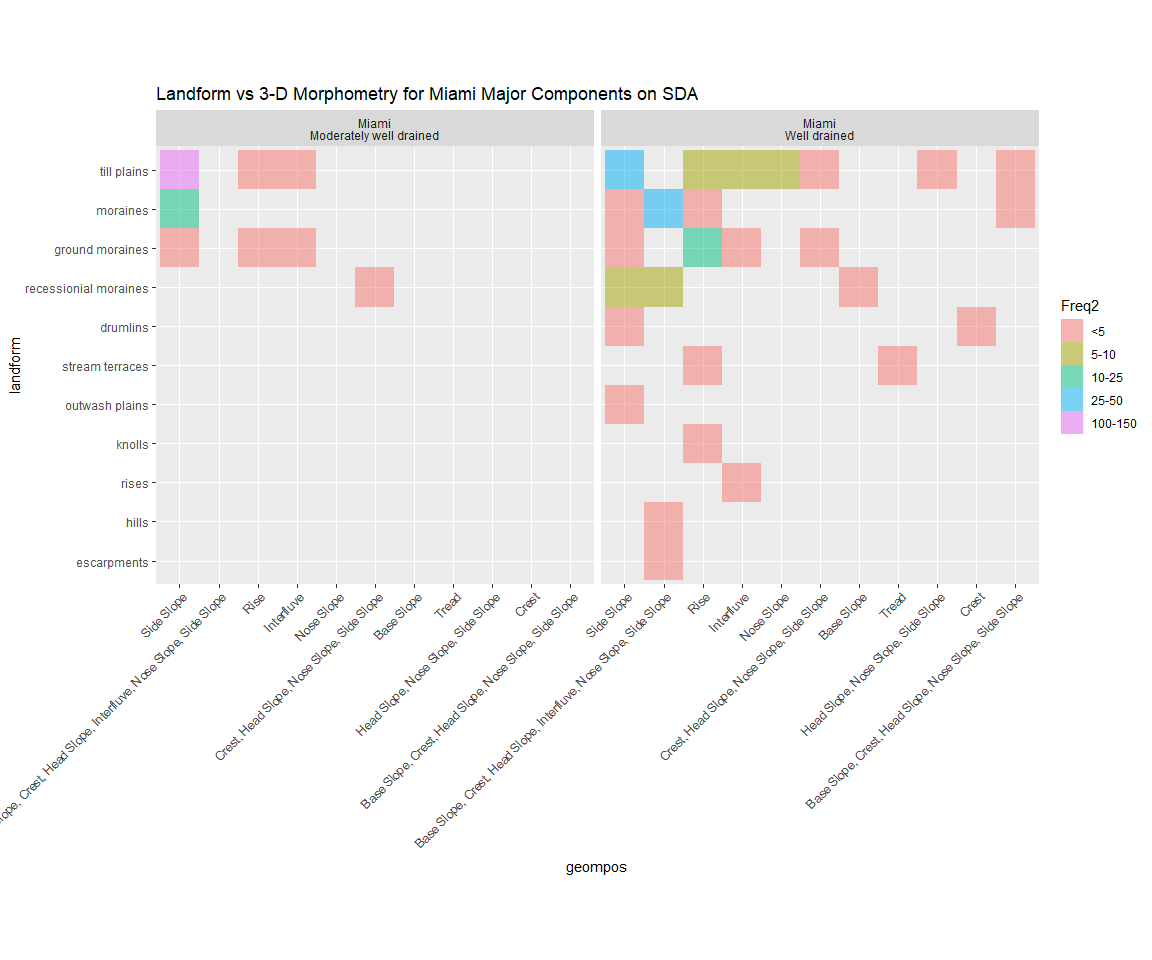# Queries Using Simple Spatial Filters

Get the map unit key and name at a single, manually-defined point (-121.77100 37.368402). Spatial queries using SDA helper functions automatically exclude STATSGO records.

``````q <- "SELECT mukey, muname
FROM mapunit
WHERE mukey IN (
SELECT * from SDA_Get_Mukey_from_intersection_with_WktWgs84('point(-121.77100 37.368402)')
)"

res <- SDA_query(q)
kable(res)``````
mukey muname
1882921 Diablo clay, 15 to 30 percent slopes, MLRA 15

Get the map names and mukey values for a 1000m buffer around a manually-defined point (-121.77100 37.368402). A 1000m buffer applied to geographic coordinates will require several spatial transformations. First, the query point needs to be initialized in a geographic coordinate system with WGS84 datum. Next, the point is transformed to a planar coordinate system with units in meters; the NLCD coordinate reference system works well for points within the continental US. After computing a buffer in planar coordinates, the resulting polygon is converted back to geographic coordinates–this is what SDA is expecting.

``````# the query point is in geographic coordinates with WGS84 datum
p <- SpatialPoints(cbind(-121.77100, 37.368402), proj4string = CRS('+proj=longlat +datum=WGS84'))
# transform to planar coordinate system for buffering
p.aea <- spTransform(p, CRS('+proj=aea +lat_1=29.5 +lat_2=45.5 +lat_0=23 +lon_0=-96 +x_0=0 +y_0=0 +ellps=GRS80 +datum=NAD83 +units=m +no_defs '))
# create 1000 meter buffer
p.aea <- gBuffer(p.aea, width = 1000)
# transform back to WGS84 GCS
p.buff <- spTransform(p.aea, CRS('+proj=longlat +datum=WGS84'))
# convert to WKT
p.wkt <- writeWKT(p.buff)

q <- paste0("SELECT mukey, muname
FROM mapunit
WHERE mukey IN (
SELECT * from SDA_Get_Mukey_from_intersection_with_WktWgs84('", p.wkt, "')
)")

res <- SDA_query(q)
mukey muname
456983 Diablo clay, 9 to 15 percent slopes
456993 Gaviota loam, 15 to 30 percent slopes
457017 Los Gatos-Gaviota complex, 50 to 75 percent slopes
1882920 Diablo clay, 30 to 50 percent slopes, MLRA 15
1882921 Diablo clay, 15 to 30 percent slopes, MLRA 15
1882923 Alo-Altamont complex, 15 to 30 percent slopes

It is possible to download small collections of SSURGO map unit polygons from SDA using an `sp` object with valid CRS. The `geomIntersection` argument to `SDA_spatialQuery()` toggles overlap (`FALSE`) vs. intersection (`TRUE`).

``````# use the buffered point geometry to query SDA
# requesting spatial intersection vs. overlapping features
p.mu.polys <- SDA_spatialQuery(p.buff, what = 'mupolygon', geomIntersection = TRUE)``````

Graphical description of the previous steps: query point, 1000m buffer, buffer bounding box (BBOX), and intersection of buffered point / map unit polygons.

``````# plot
par(mar=c(0,0,0,0))
plot(p.mu.polys)
lines(p.buff, col='red', lwd=2)
points(p, col='orange', pch=15)
legend('bottomleft', legend=c('query point', '1000m buffer', 'buffer BBOX', 'intersected polygons'), col=c('orange', 'red', 'royalblue', 'black'), lwd=c(NA, 2, 2, 2), pch=c(15, NA, NA, NA))
box()``````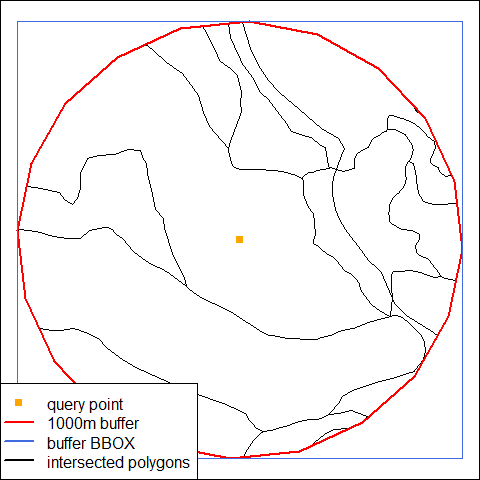Get some component data for a manually-defined bounding box, defined in WGS84 geographic coordinates.

``````# define a bounding box: xmin, xmax, ymin, ymax
#
#         +-------------------(ymax, xmax)
#         |                        |
#         |                        |
#     (ymin, xmin) ----------------+

b <- c(-120.9, -120.8, 37.7, 37.8)
# convert bounding box to WKT
p <- writeWKT(as(extent(b), 'SpatialPolygons'))
# compose query, using WKT BBOX as filtering criteria
q <- paste0("SELECT mukey, cokey, compname, comppct_r
FROM component
WHERE mukey IN (SELECT DISTINCT mukey FROM SDA_Get_Mukey_from_intersection_with_WktWgs84('", p, "') )
ORDER BY mukey, cokey, comppct_r DESC")

res <- SDA_query(q)

# check
mukey cokey compname comppct_r
462527 21830876 Alamo 85
462527 21830877 San Joaquin 5
462541 21831125 Chualar 85
462541 21831126 Oakdale 5
462541 21831127 Modesto 5

# Queries Using Complex Spatial Filters

The examples above illustrate how to perform SDA queries using a single spatial filter. Typically we need to perform these queries over a collection of points, lines or polygons. The `soilDB` package provides some helper functions for iterating over a collection of features (usually points).

TODO: document `SDA_query_features` vs. `SDA_spatialQuery`

The function `SDA_spatialQuery()` will convert `Spatial*` (Points, Lines, Polygons) objects to WKT and submit a GeometryCollection to SDA. Depending on the argument `what`, the function will return intersecting map unit polygons and keys (`what=geom`) or just the mukey and map unit name (`what=mukey`). The latter mode is much faster than the former.

An example based on a `SpatialPoints` object with 1 feature, asking for only tabular results.

``````# the query point is in geographic coordinates with WGS84 datum
p <- SpatialPoints(cbind(-121.77100, 37.368402), proj4string = CRS('+proj=longlat +datum=WGS84'))

# p is a spatial filter applied to a query against the map unit polygons
# results contain intersecting map unit keys and names
res <- SDA_spatialQuery(p, what = 'mukey')
kable(res)``````
mukey muname
1882921 Diablo clay, 15 to 30 percent slopes, MLRA 15

Again with the same data, this time asking for spatial results.

``````# results contain intersecting polygons and their map unit keys
res <- SDA_spatialQuery(p, what = 'geom')

# check graphically
par(mar=c(1,1,1,1))
plot(res)
points(p, bg='royalblue', pch=21, cex=2)
box()``````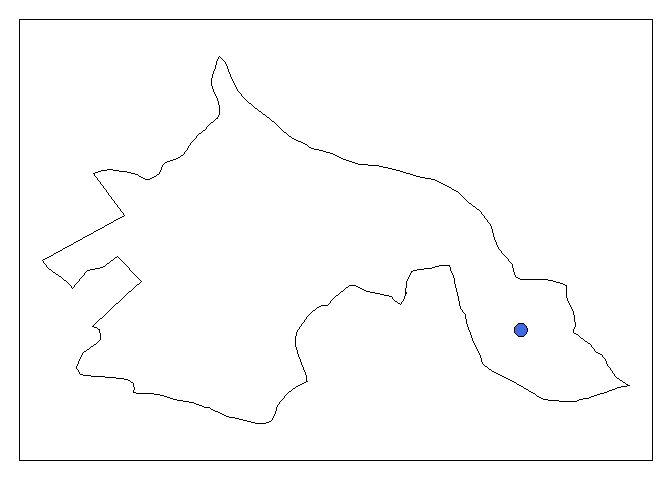Some things to consider when using `SDA_spatialQuery()`:

• spatial filters must have a valid CRS defined
• spatial queries for more than 1000 features should probably be done using a local copy of the map unit polygons
• order is not preserved
• the number of resulting rows will not likely match the number of source rows
• results must be joined to the source data via spatial intersection, e.g. `sp::over()`

Let’s apply the `SDA_spatialQuery()` function to some real data; KSSL pedons correlated to “Auburn”. Not all of these pedons have coordinates, so we will have to do some filtering first. See the in-line comments for details on each line of code.

``````# get KSSL pedons with taxonname = Auburn
# coordinates will be WGS84 GCS
auburn <- fetchKSSL('auburn')
# keep only those pedons with valid coordinates
auburn <- subsetProfiles(auburn, s='!is.na(x) & !is.na(y)')
# init spatial information
coordinates(auburn) <- ~ x + y
# define projection
proj4string(auburn) <- '+proj=longlat +datum=WGS84'

# extract "site data"
auburn.sp <- as(auburn, 'SpatialPointsDataFrame')

# perform SDA query on collection of points
mu.data <- SDA_spatialQuery(auburn.sp, what = 'geom')

# use local spatial intersection to link source + SDA data
auburn.sp\$mukey <- over(auburn.sp, mu.data)\$mukey

# join results to original SoilProfileCollection using 'pedlabsampnum'
site(auburn) <- as.data.frame(auburn.sp)[, c('pedlabsampnum', 'mukey')]``````

Check the results and plot the “Auburn” KSSL pedons, grouped by intersecting map unit key and coloring horizons according to clay content.

``````# plot profiles, grouped by mukey
# color horizons with clay content
par(mar=c(0,2,4,0))
groupedProfilePlot(auburn, groups='mukey', group.name.cex=0.65, color='clay', name='hzn_desgn', id.style='side', label='pedon_id', max.depth=100)
# describe IDs
mtext('user pedon ID', side=2, line=-1.5)
mtext('mukey', side=3, line=-1, at = c(0,0), adj = 0)``````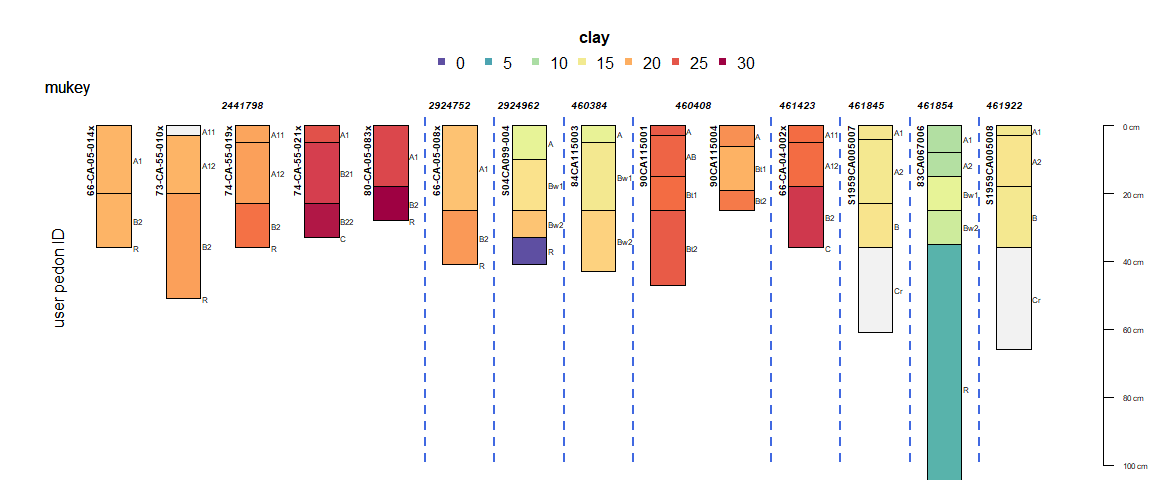This document is based on `soilDB` version 2.6.5.